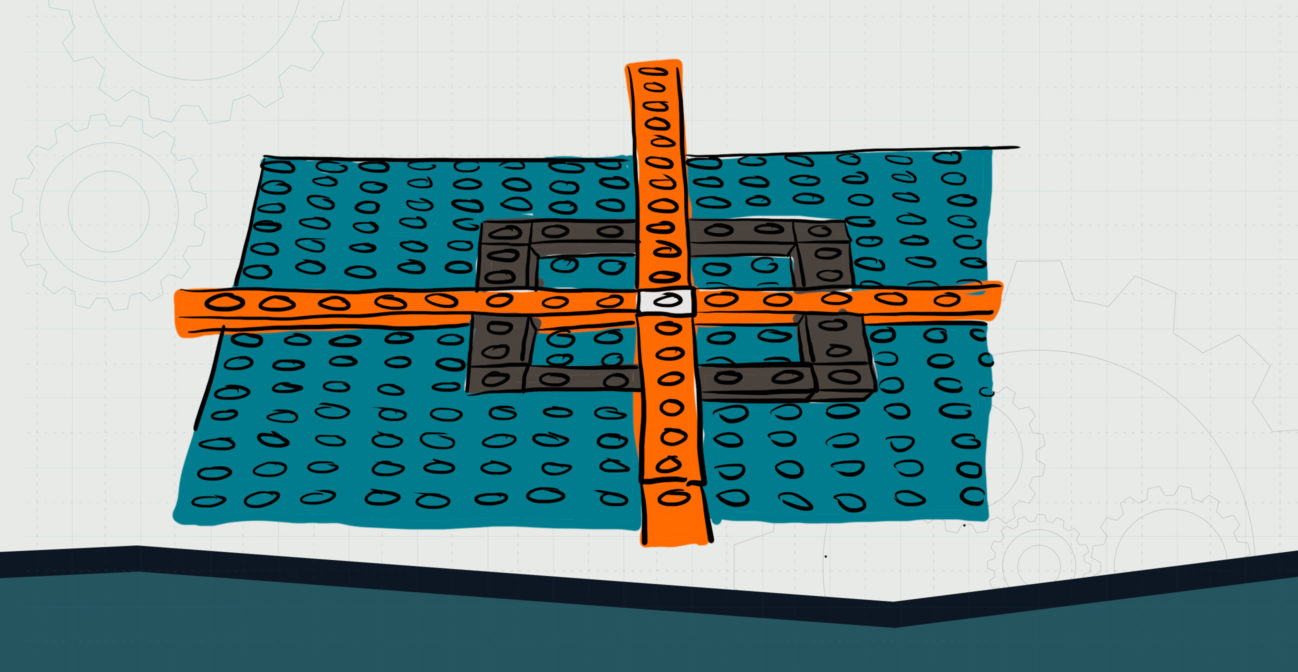# Why Coordinate?

## Use plotting to design polygons and study their properties.Objective:
Child will be able to plot and draw polygons on a coordinate plane given a set of vertices. They will be able to determine side lengths using their graphs.

Essential Question:
How might we represent polygons and measure sides using coordinate planes?

Special Materials:
None

Bricks Required:
1x bricks of various sizes; two brick plates

Project Structure

Engage/Explain:

1. Have child create a coordinate plane using SOHO Bricks.
2. Provide them a set of vertices to represent a square: (-3,3) (3,3) (3,-3) (-3,-3)
3. Ask child to identify the polygon shape they see.
1. If child has trouble visualizing the shape, they may connect the lines from point to point using a different color brick.

Explore:

1. Ask child to determine the length of the side and explain how they got their answer.
1. Most children will probably count the studs to determine their answers; be careful to facilitate thinking on counting so they arrive at the correct answers.
2. Ask child if they can devise a “formula” for determining the correct length using the coordinates.
1. Provide time for child to explore various formulas of their creation, always checking the correct answer to see if they match.
2. The equation they should arrive at is the absolute difference between the two coordinates (i.e. |x2-x1|).

Explain/Elaborate:

1. Provide child additional paper practice problems to determine polygon shapes and length of sides.